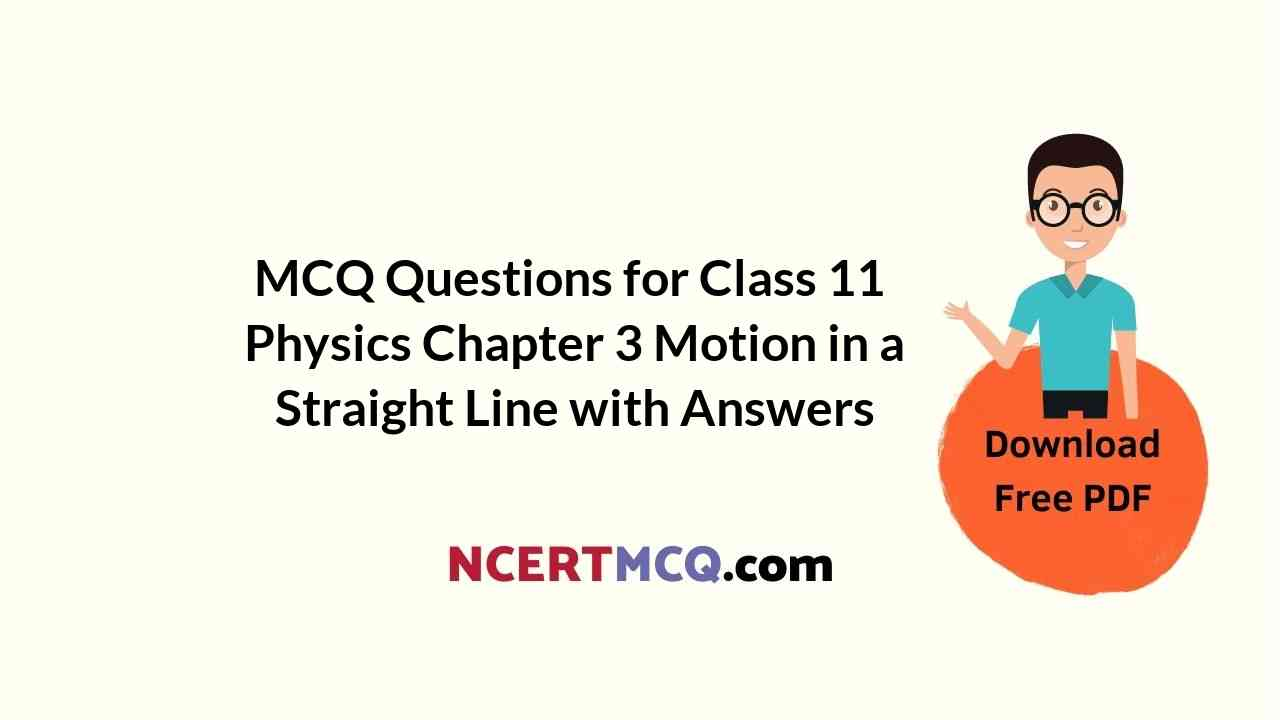Check the below NCERT MCQ Questions for Class 11 Physics Chapter 3 Motion in a Straight Line with Answers Pdf free download. MCQ Questions for Class 11 Physics with Answers were prepared based on the latest exam pattern. We have provided Motion in a Straight Line Class 11 Physics MCQs Questions with Answers to help students understand the concept very well.

## Motion in a Straight Line Class 11 MCQs Questions with Answers

Motion In A Straight Line Class 11 MCQ Question 1.
The displacement in metres of a body varies with time t in second as y = t2 – t – 2. The displacement is zero for a positive of t equal to
(a) 1 s
(b) 2 s
(c) 3 s
(d) 4 s

Class 11 Physics Chapter 3 MCQ Question 2.
A boy starts from a point A, travels to a point B at a distance of 3 km from A and returns to A. If he takes two hours to do so, his speed is
(a) 3 km/h
(b) zero
(c) 2 km/h
(d) 1.5 km/h

Motion In A Straight Line MCQ Question 3.
A 180 metre long train is moving due north at a speed of 25 m/s. A small bird is flying due south, a little above the train, with a speed of 5 m/s. The time taken by the bird to cross the train is
(a) 10 s
(b) 12 s
(c) 9 s
(d) 6 s

MCQ Questions For Class 11 Physics Chapter 3 Question 4.
A boy starts from a point A, travels to a point B at a distance of 1.5 km and returns to A. If he takes one hour to do so, his average velocity is
(a) 3 km/h
(b) zero
(c) 1.5 km/h
(d) 2 km/h

Motion In A Straight Line Class 11 MCQs Question 5.
A body starts from rest and travels with uniform acceleration on a straight line. If its velocity after making a displacement of 32 m is 8 m/s, its acceleration is
(a) 1 m/s²
(b) 2 m/s²
(c) 3 m/s²
(d) 4 m/s²

Class 11 Physics Chapter 3 MCQ With Answers Question 6.
Which one of the following is the unit of velocity?
(a) kilogram
(b) metre
(c) m/s
(d) second

Motion In Straight Line Class 11 MCQ Question 7.
A body starts from rest and travels for t second with uniform acceleration of 2 m/s². If the displacement made by it is 16 m, the time of travel t is
(a) 4 s
(b) 3 s
(c) 6 s
(d) 8 s

Ch 3 Physics Class 11 MCQ Question 8.
The dimensional formula for speed is
(a) T-1
(b) LT-1
(c) L-1T-1
(d) L-1T

Class 11 Physics Ch 3 MCQ Question 9.
The dimensional formula for velocity is
(a) [LT]
(b) [LT-1]
(c) [L2T]
(d) [L-1T]

Motion In Straight Line MCQ Question 10.
A body starts from rest and travels with an acceleration of 2 m/s². After t seconds its velocity is 10 m/s . Then t is
(a) 10 s
(b) 5 s
(c) 20 s
(d) 6 s

Class 11 Motion In A Straight Line MCQ Question 11.
A boy starts from a point A, travels to a point B at a distance of 1.5 km and returns to A. If he takes one hour to do so, his average velocity is
(a) 3 km/h
(b) zero
(c) 1.5 km/h
(d) 2 km/h

Chapter 3 Physics Class 11 MCQ Question 12.
A body starts from rest. If it travels with an acceleration of 2 m/s², its displacement at the end of 3 seconds is
(a) 9 m
(b) 12 m
(c) 16 m
(d) 10 m

Physics Class 11 Chapter 3 MCQ Question 13.
A body starts from rest and travels with uniform acceleration of 2 m/s². If its velocity is v after making a displacement of 9 m, then v is
(a) 8 m/s
(b) 6 m/s
(c) 10 m/s
(d) 4 m/s

Chapter 3 Physics Class 11 MCQs Question 14.
A body starts from rest and travels with an acceleration of 2 m/s². After t seconds its velocity is 10 m/s. Then t is
(a) 10 s
(b) 5 s
(c) 20 s
(d) 6 s

MCQ Of Ch 3 Physics Class 11 Question 15.
A body starts from rest and travels for five seconds to make a displacement of 25 m if it has travelled the distance with uniform acceleration a then a is
(a) 3 m/s²
(b) 4 m/s²
(c) 2 m/s²
(d) 1 m/s²

MCQ On Motion In A Straight Line Question 16:
A boy moves on a circular distance of radius R. Starting from a point A he moves to a point B which is on the other end of the diameter AB. The ratio of the distance travelled to the displacement made by him is
(a) ∏/2
(b) ∏
(c) 2∏
(d) 4∏

Class 11 Physics Motion In A Straight Line MCQ With Answers Question 17.
The dimensional formula for acceleration is
(a) [LT2]
(b) [LT?2]
(c) [L2T]
(d) [L2T2]

MCQ Of Chapter 3 Physics Class 11 Question 18.
A body starts from rest and travels with uniform acceleration a to make a displacement of 6 m. If its velocity after making the displacement is 6 m/s, then its uniform acceleration a is
(a) 6 m/s²
(b) 2 m/s²
(c) 3 m/s²
(d) 4 m/s²

MCQ Class 11 Physics Chapter 3 Question 19.
Which one of the following is the unit of velocity?
(a) kilogram
(b) metre
(c) m/s
(d) second

Physics Chapter 3 MCQ Class 11 Question 20.
The displacement in metres of a body varies with time t in second as y = t2 – t – 2. The displacement is zero for a positive of t equal to
(a) 1 s
(b) 2 s
(c) 3 s
(d) 4 s